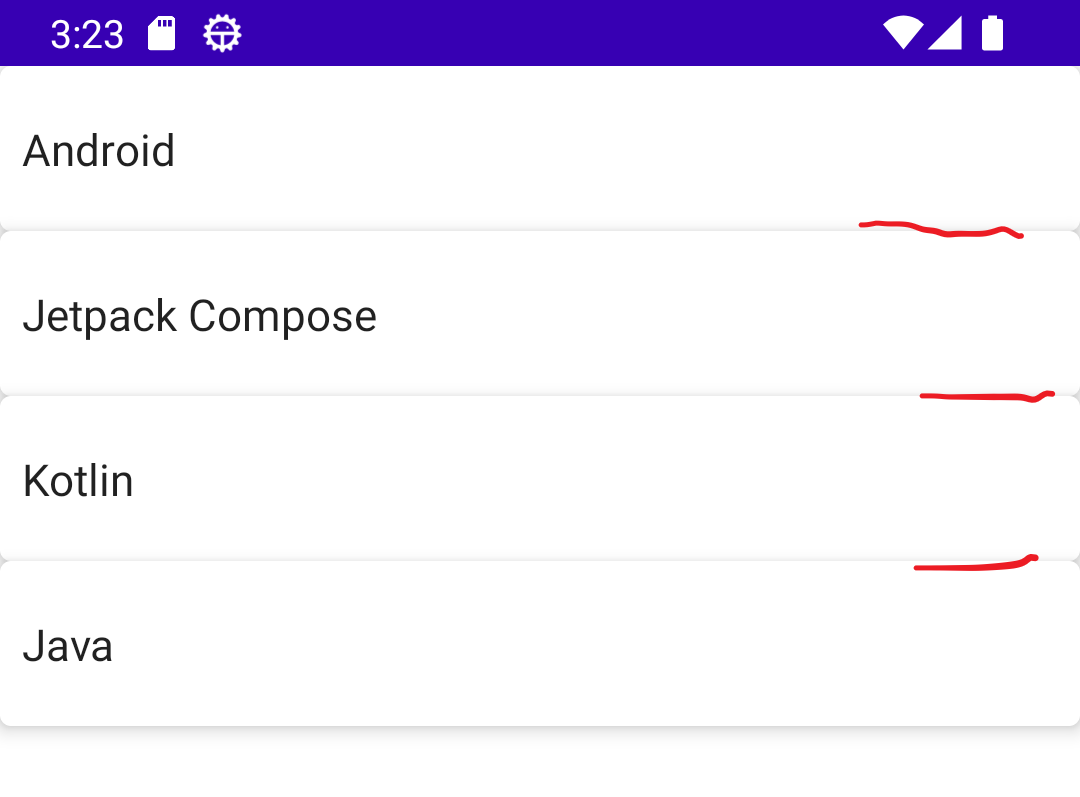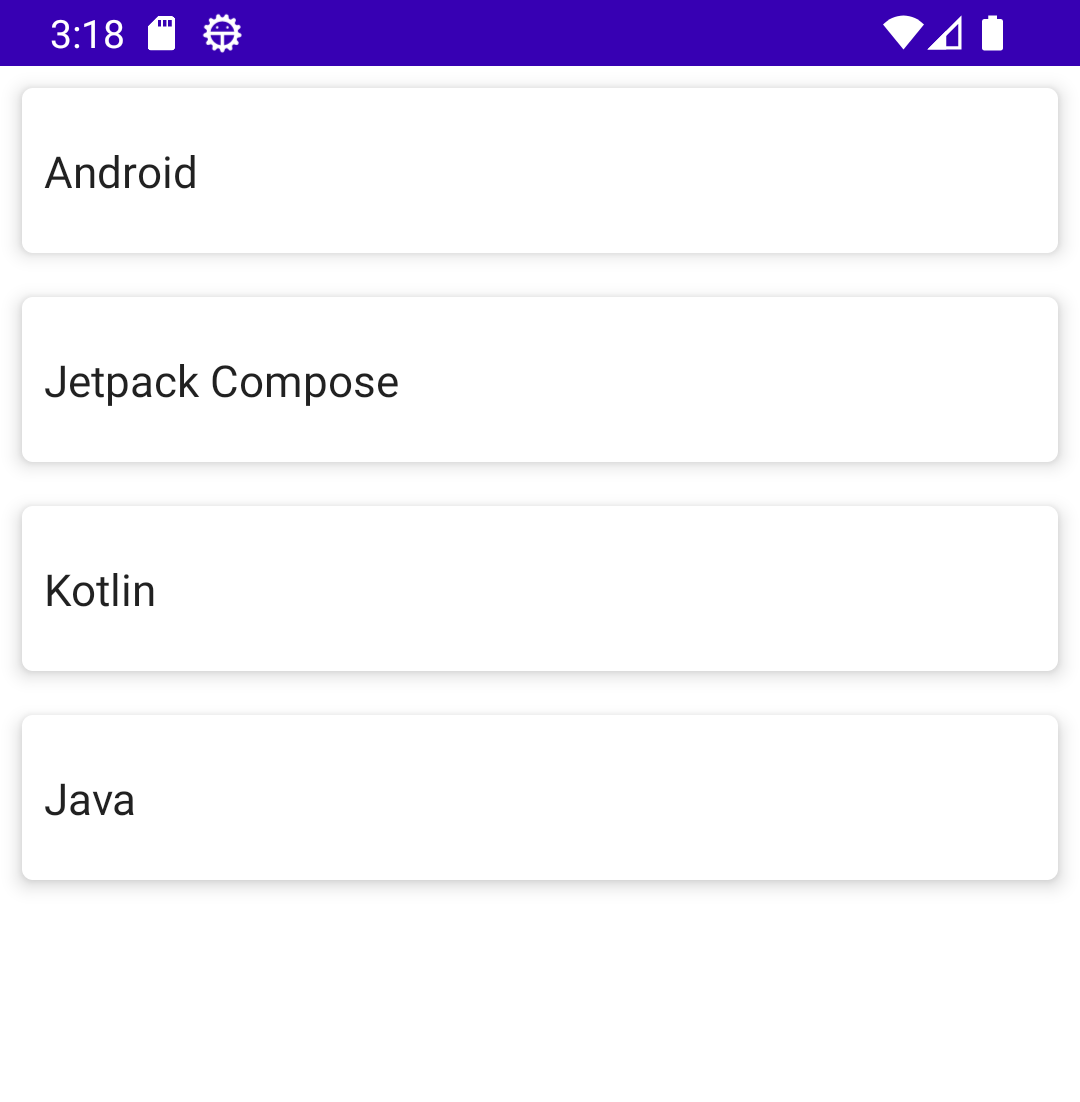# How can i add margin to compose widgets?

Published August 05, 2022

While create android UI with xmls we have option to set gap between widgets by adding margin properties. Example marginLeft, marginRight,marginTop and marginBottom. Where as while design with compose widgets there is no direct way to add margins to widgets. To get gap between widgets we will apply modifier properties to the respected widget and get the margins.

Let see We have multiple cards inside Column widget

To create new Jetpack project in Android studio please refer how to create new Project in Android studio using Jetpack compose

 @Composable fun displayCards() {     Column() {         Card(             elevation = (5f.dp),                     ) {             Row( modifier = Modifier.fillMaxWidth().height(60.dp).padding(all = 8.dp), verticalAlignment = Alignment.CenterVertically) {                 Text(text = "Android")             }         }         Card(             elevation = (5f.dp),                     ) {             Row(  modifier = Modifier.fillMaxWidth().height(60.dp).padding(all = 8.dp), verticalAlignment = Alignment.CenterVertically) {                 Text(text = "Jetpack Compose")             }         }         Card(             elevation = (5f.dp),                     ) {             Row(  modifier = Modifier.fillMaxWidth().height(60.dp).padding(all = 8.dp), verticalAlignment = Alignment.CenterVertically) {                 Text(text = "Kotlin")             }         }         Card(             elevation = (5f.dp),                     ) {             Row( modifier = Modifier.fillMaxWidth().height(60.dp).padding(all = 8.dp), verticalAlignment = Alignment.CenterVertically) {                 Text(text = "Java")             }         }     } }

While run the above code it will return below screenThere is no gap between cards. Let see how to add margins to Card widget using Modifier padding properties
To add margins to card we are applying the modifier to the card with padding properties

So this will create gap between the cards

Now run the application you will see the below ui which contains gap between the card widgets inside column widgets@Composable fun displayCards() {     Column() {         Card(             elevation = (5f.dp),             modifier = Modifier                 .padding(8.dp)         ) {             Row( modifier = Modifier.fillMaxWidth().height(60.dp).padding(all = 8.dp), verticalAlignment = Alignment.CenterVertically) {                 Text(text = "Android")             }         }         Card(             elevation = (5f.dp),             modifier = Modifier                 .padding(8.dp)         ) {             Row(  modifier = Modifier.fillMaxWidth().height(60.dp).padding(all = 8.dp), verticalAlignment = Alignment.CenterVertically) {                 Text(text = "Jetpack Compose")             }         }         Card(             elevation = (5f.dp),             modifier = Modifier                 .padding(8.dp)         ) {             Row(  modifier = Modifier.fillMaxWidth().height(60.dp).padding(all = 8.dp), verticalAlignment = Alignment.CenterVertically) {                 Text(text = "Kotlin")             }         }         Card(             elevation = (5f.dp),             modifier = Modifier                 .padding(8.dp)         ) {             Row( modifier = Modifier.fillMaxWidth().height(60.dp).padding(all = 8.dp), verticalAlignment = Alignment.CenterVertically) {                 Text(text = "Java")             }         }     } }

Conclusion: In this compose example we have implemented how to add margins to the card widgets

 Article Contributed By :160 Views
Projects By category
Categories